Textbook 6B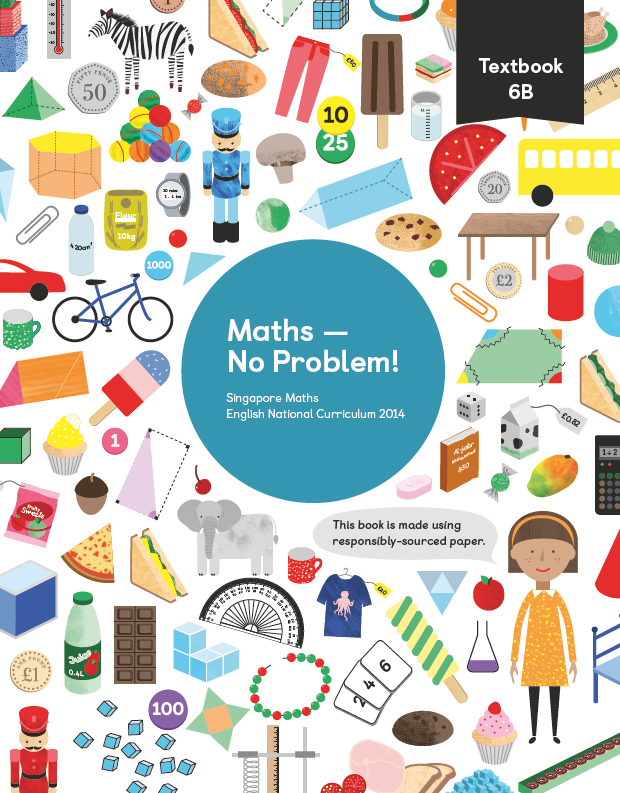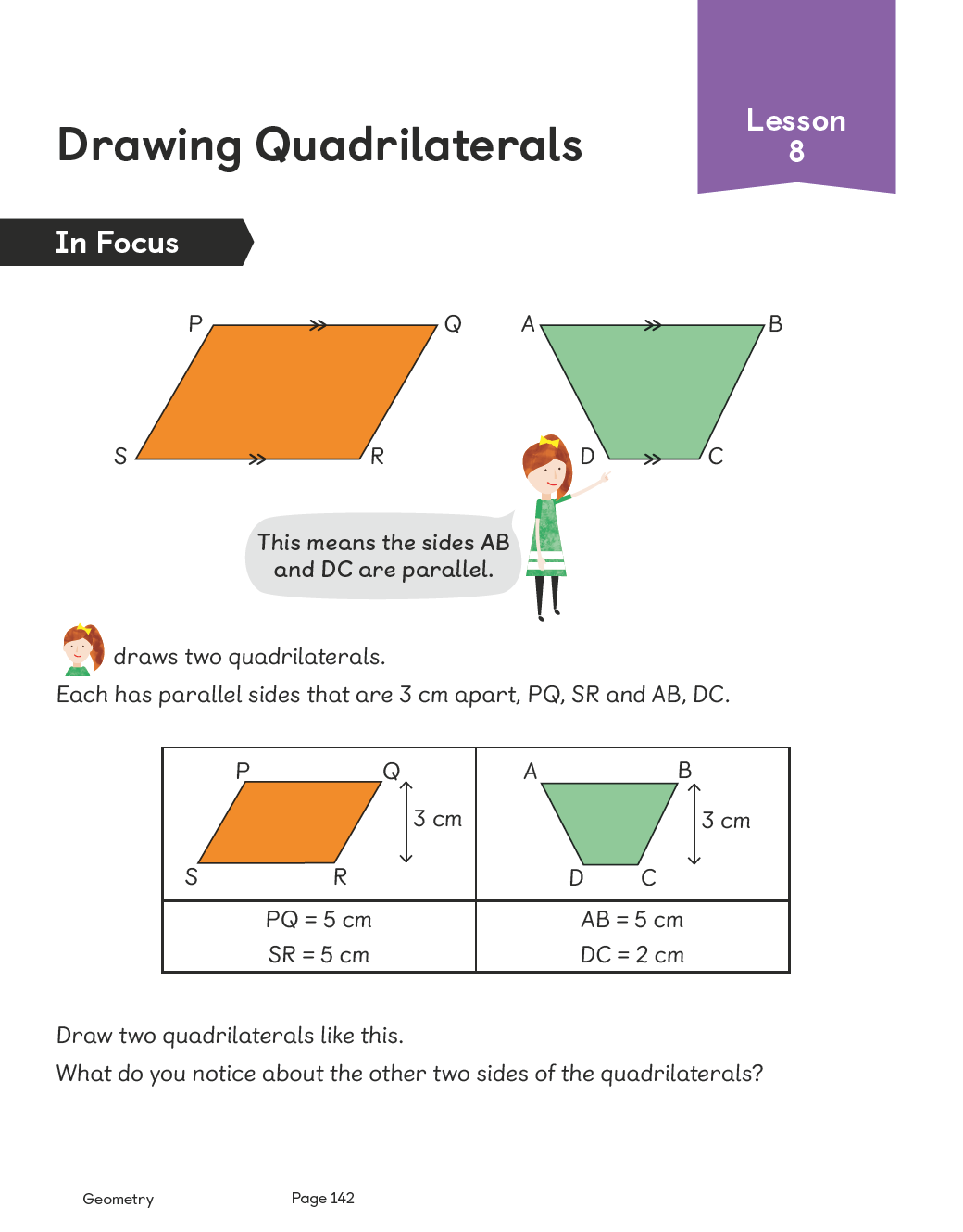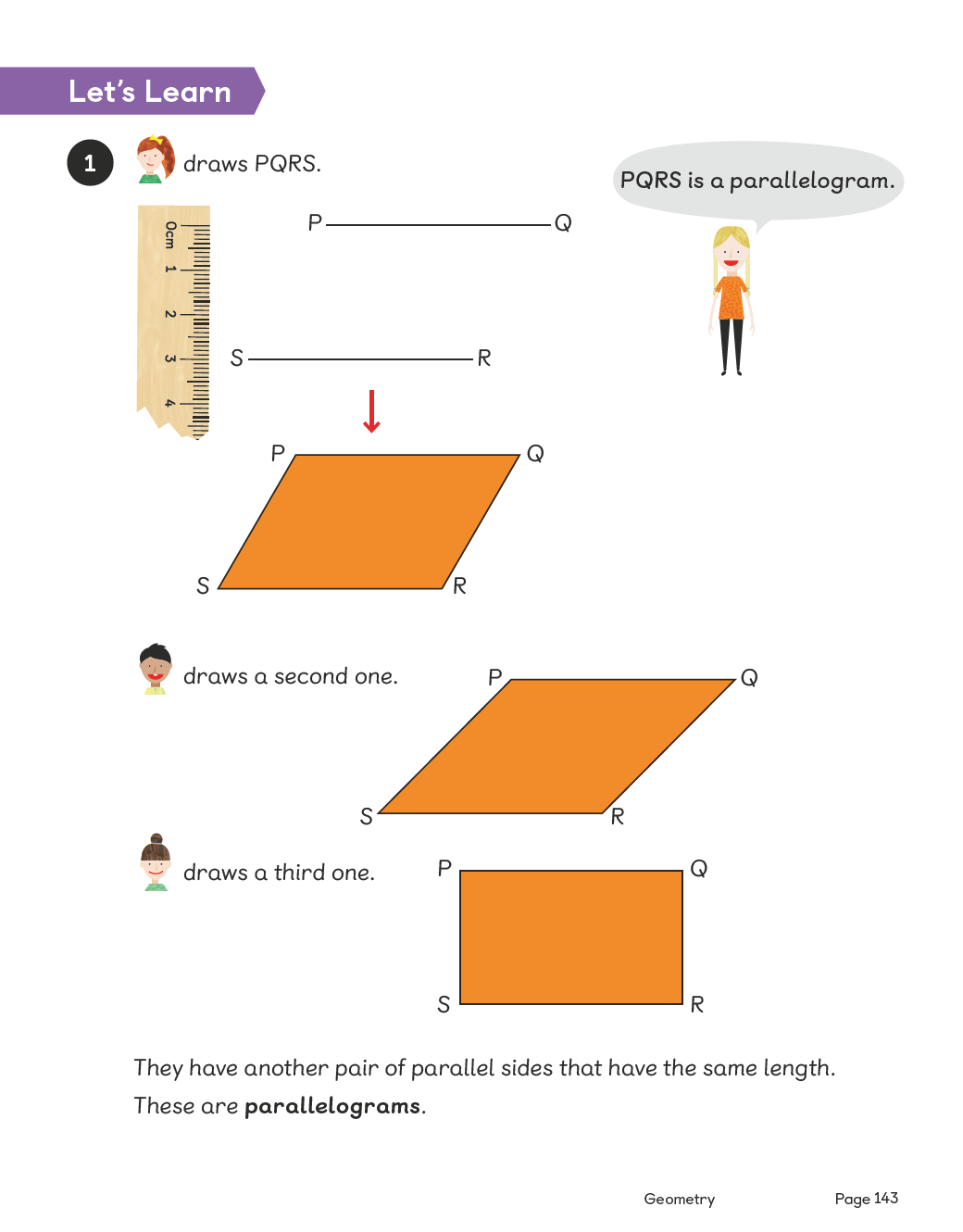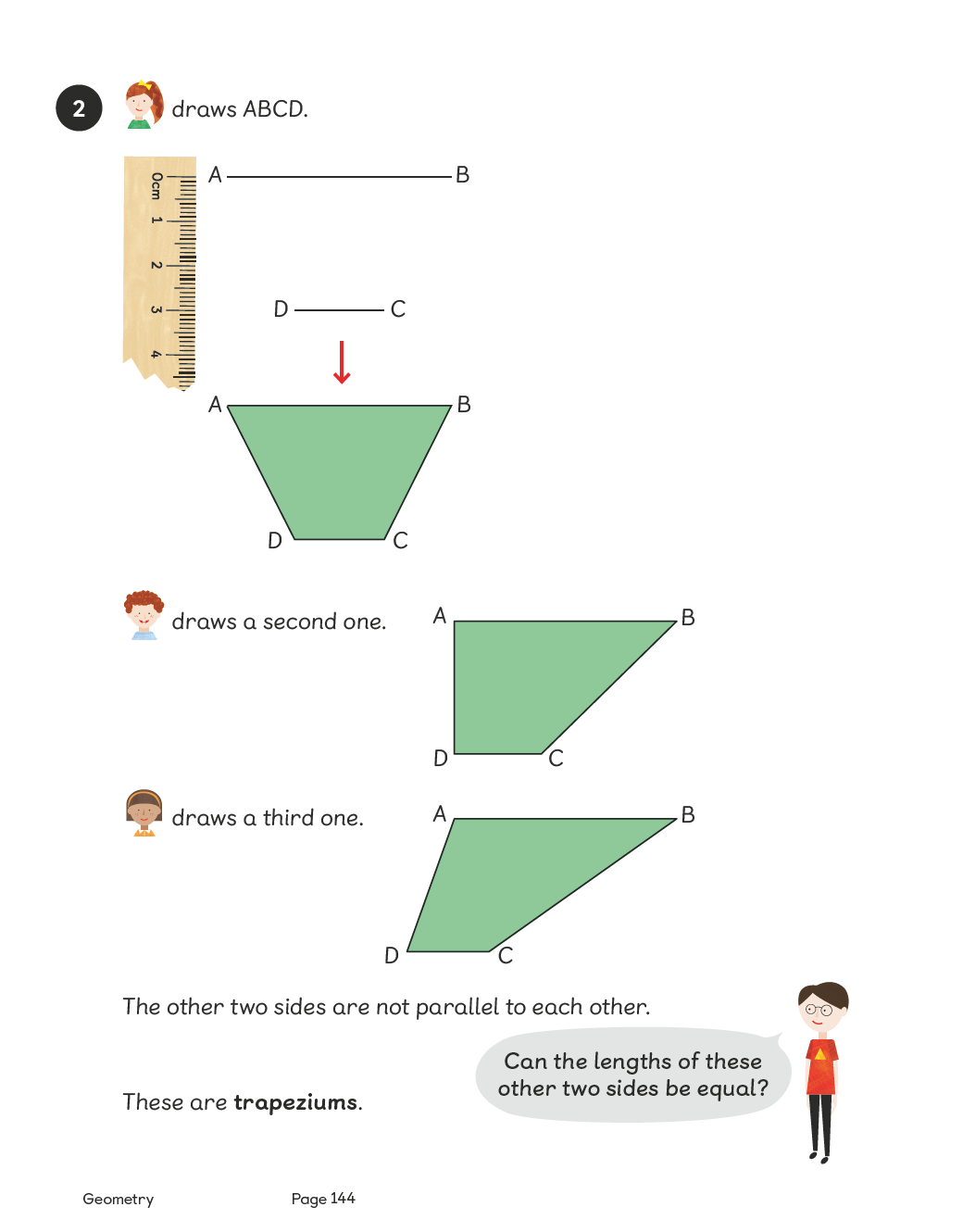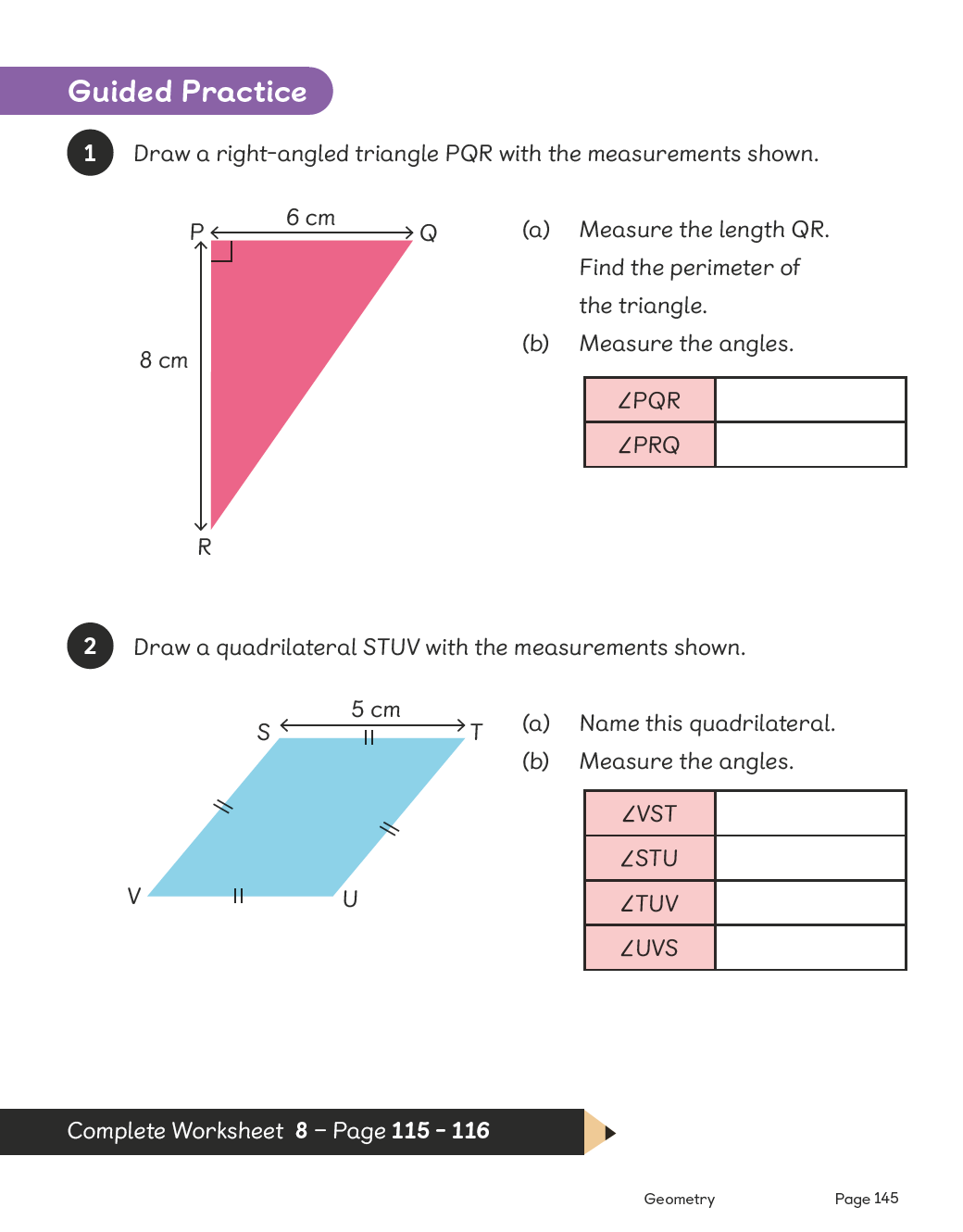# Maths — No Problem!Textbook 6B

## £9.99

ISBN: 9781910504222
Pages: 266
Availability:
In stock
Qty:

• Lesson 1 - Finding the Percentage of a Number

To be able to calculate percentages of a whole number.

• Lesson 2 - Finding the Percentage of a Quantity

To be able to calculate percentages of a quantity.

• Lesson 3 - Finding Percentage Change

To be able to solve problems involving the calculation of percentages.

• Lesson 4 - Using Percentage to Compare

To be able to use percentage for comparison. To be able to use equivalences between simple fractions, decimals and percentages in different contexts.

• Lesson 5 - Chapter Consolidation

To be able to use knowledge of percentage to solve problems.

## Author(s)

Consultant and Author: Dr. Yeap Ban Har
UK Consultant: Dr. Anne Hermanson